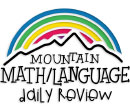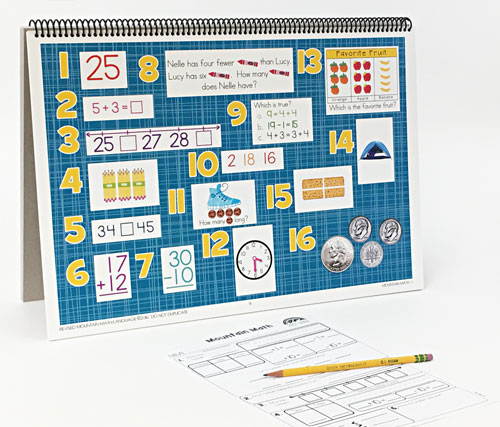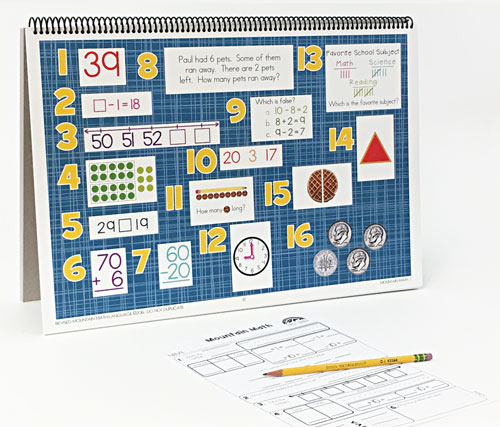WHAT YOU GET:
• 32 WEEKS OF DAILY REVIEW
• INSTRUCTIONS
• ONE MASTER STUDENT RESPONSE SHEET
• HEAVY DUTY STAND
• KNOWLEDGEABLE STAFF FOR ANY ASSISTANCE

This 1st GRADE MOUNTAIN MATH CENTER EDITION is the DAILY REVIEW your students need to MASTER the concepts you have taught.

Research has proven that LONG-TERM MASTERY comes from SPIRAL REVIEW, also called DISTRIBUTED PRACTICE AND SPACED REPETITION.

The CENTER EDITION is designed for small group review, such as rotations, scoots, centers, or math-around-the-room. It is a smaller version (13"x19") of our Bulletin Board Kit. It can easily be set up and taken down as needed.
1. Generate a number that is greater than or less than a given whole number up to 120.
Use place value to compare whole numbers up to 120 using comparative language.
Use relationships to determine the number that is 10 more and 10 less than a given number up to 120.
2. Use objects and pictorial models to solve word problems involving joining, separating, and comparing sets within 20 and unknowns as any one of the terms in the problem such as 2 + 4 = [ ]; 3 + [ ] = 7; and 5 = [ ] - 3.
Determine the unknown whole number in an addition or subtraction equation when the unknown may be any one of the three or four terms in the equation.
Understand that the equal sign represents a relationship where expressions on each side of the equal sign represent the same value(s).
3. Order whole numbers up to 120 using place value and open number lines.
Recite numbers forward and backward from any given number between 1 and 120.
4. Recognize instantly the quantity of structured arrangements.
Use concrete and pictorial models to compose and decompose numbers up to 120 in more than one way as so many hundreds, so many tens, and so many ones.
Use objects, pictures, and expanded and standard forms to represent numbers up to 120.
Use concrete and pictorial models to determine the sum of a multiple of 10 and a one-digit number in problems up to 99.
5. Represent the comparison of two numbers to 100 using the symbols >, <, or =.
6. Compose 10 with two or more addends with and without concrete objects.
Apply properties of operations to add and subtract two or three numbers.
7. Compose 10 with two or more addends with and without concrete objects.
Apply properties of operations to add and subtract two or three numbers.
8. Explain strategies used to solve addition and subtraction problems up to 20 using spoken words, objects, pictorial models, and number sentences.
Generate and solve problem situations when given a number sentence involving addition or subtraction of numbers within 20.
Represent word problems involving addition and subtraction of whole numbers up to 20 using concrete and pictorial models and number sentences.
9. Understand that the equal sign represents a relationship where expressions on each side of the equal sign represent the same value(s).
10. Apply basic fact strategies to add and subtract within 20, including making 10 and decomposing a number leading to a 10.
Explain strategies used to solve addition and subtraction problems up to 20 using spoken words, objects, pictorial models, and number sentences.
11. Use measuring tools to measure the length of objects to reinforce the continuous nature of linear measurement.
Illustrate that the length of an object is the number of same-size units of length that, when laid end-to-end with no gaps or overlaps, reach from one end of the object to the other.
Measure the same object/distance with units of two different lengths and describe how and why the measurements differ.
12. Tell time to the hour and half hour using analog and digital clocks.
13. Collect, sort, and organize data in up to three categories using models/representations such as tally marks or T-charts.
Use data to create picture and bar-type graphs.
Draw conclusions and generate and answer questions using information from picture and bar-type graphs.
14. Classify and sort regular and irregular two-dimensional shapes based on attributes using informal geometric language.
Distinguish between attributes that define a two-dimensional or three-dimensional figure and attributes that do not define the shape.
Identify two-dimensional shapes, including circles, triangles, rectangles, and squares, as special rectangles, rhombuses, and hexagons and describe their attributes using formal geometric language.
Identify three-dimensional solids, including spheres, cones, cylinders, rectangular prisms (including cubes), and triangular prisms, and describe their attributes using formal geometric language.
15. Partition two-dimensional figures into two and four fair shares or equal parts and describe the parts using words.
Identify examples and non-examples of halves and fourths.
16. Identify U.S. coins, including pennies, nickels, dimes, and quarters, by value and describe the relationships among them.
Write a number with the cent symbol to describe the value of a coin.
Use relationships to count by twos, fives, and tens to determine the value of a collection of pennies, nickels, and/or dimes.
TEXAS ESSENTIAL KNOWLEDGE AND SKILLS CORRELATION
1. 1.2BCDE, 1.3E, 1.5ACG
2. 1.3BE, 1.5FG
3. 1.2F
4. 1.2ABC, 1.3E, 1.5CG
5. 1.2G
6. 1.5G
7. 1.5G
8. 1.3BEF, 1.5DG
9. 1.3E, 1.5EG
10. 1.3CE, 1.5G
11. 1.7ABCD
12. 1.7E
13. 1.8ABC
14. 1.6ABDE
15. 1.6GH
16. 1.4ABC
ONLINE REVIEW VS.
CENTER EDITION VS.
BULLETIN BOARD KIT
ONLINE REVIEW
Is projected onto an interactive white board, creating a virtual board. It can be viewed by the entire classroom. Answers can be displayed onto the board at the click of a button. Up to seven questions can be isolated and magnified.

CENTER EDITION
Is organized on a 13"x19" flip chart. It can be viewed by 5-6 students at a time. It was designed for small group review, such as rotations, scoots, centers, or math-around-the-classroom. It has an answer key, heavy-duty stand, and high-gauged coil.

BULLETIN BOARD KIT
It is designed for total classroom view. It hangs on bulletin boards, walls, white boards, pocket charts, and science display boards. Once hung, it can remain displayed for the entire year. The cards can be interchanged, creating flexible and adjustable DAILY REVIEW.
\$99.95 QTY: